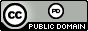시간 제한메모리 제한제출정답맞힌 사람정답 비율
2 초 512 MB16141285.714%

## 문제

Every (positive) rational number can be expressed as a ratio of two (positive) integers. However, in decimal form, rational numbers often have an infinitely repeating pattern, e.g., 1/7 = 0.142857142857142857... A convenient way of writing this repeating pattern is to put a bar over the first occurrence of the repeating part, so 1/7 would be written:

0.142857.

Given a rational number consisting of a series of digits, followed by a decimal point, followed by more digits, and then a number indicating how many of the rightmost digits repeat (i.e., the number of digits under the bar), your task is to find the ratio of two integers, in the most reduced form, that represent the same rational number. For example, for the input “0.142857 6” you should find 1/7.

## 입력

The input will be a single line with two numbers separated by one space. The first number will consist of 1 to 3 digits (0–9), followed by a decimal point, followed by 1 to 11 digits (0–9), representing the decimal form of the number, possibly with leading zeros. The second number will be a positive integer indicating how many of the rightmost digits of the preceding number repeat. The first number will always be greater than 0. The second number will never be less than 1 nor larger than the number of digits to the right of the decimal point.

## 출력

Print the corresponding fraction in its most reduced form, that is, the fraction with the smallest possible integer values in the numerator and denominator.

## 예제 입력 1

0.142857 6


## 예제 출력 1

1/7


## 예제 입력 2

1.6 1


## 예제 출력 2

5/3


## 예제 입력 3

123.456 2


## 예제 출력 3

61111/495


## 출처• 문제를 만든 사람: David Poplawski